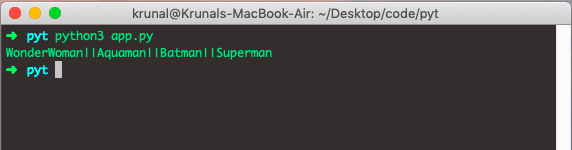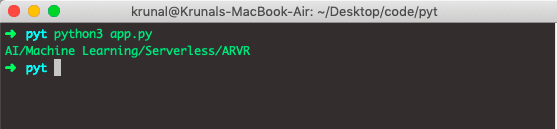# Python String join: The Complete Guide

Python join function provides a flexible way to concatenate a string. It concatenates each element of an iterable (such as a list, string, and a tuple) to the string and returns the concatenated string.

## Python Join Example

The string join() is a built-in Python function that returns the string concatenated with the elements of an iterable. The join() method is the string method that returns the string in which the elements of a sequence have been joined by a string separator.

### Syntax

The syntax of the join() method is the following.

string.join(iterable)

### Parameters

Python String join() method takes required iterable, which are objects capable of returning its members one at a time. Some examples are List, Tuple, String, Dictionary, and Set.

If the iterable contains any non-string values, it raises a TypeError exception.

See the following example. Let’s define a tuple and then join that tuple values with the and see the output.

# app.py

venom = ('Knull', 'Toxin', "Carnage")
single = '|'.join(venom)
print(single)

See the output.## #Python join() method in the list

Strings in Python are immutable, and so ‘string X’ + ‘string Y’  has to make a third-string to combine them.

Say you want to clone the string, by adding each item to the string will get ?(?2)O(n2) time, as opposed to ?(?)O(n) as you would get if it were a list and the best way to join an iterable by the separator is to use str.join().

Let’s define a list and then join the items with the join method with a separator.

# app.py

DC = ['WonderWoman', 'Aquaman', 'Batman', 'Superman']
movies = '||'.join(DC)
print(movies)


Okay, so we have defined a list and then join with a separator || and now see the output.If you want to do this manually, then I’d accept the ?(?2)O(n2) performance, and write something easy to understand.

One way to do this is to take the first element and add the separator and an item every time after, such as:

See the following code.

def join(iterator, seperator):
it = map(str, iterator)
seperator = str(seperator)
string = next(it, '')
for s in it:
string += seperator + s
return string



## #Python join() method in Dictionary

Let’s define a dictionary and then join the items with the join method with a separator.

# app.py

trends = {
1: 'AI',
2: 'Machine Learning',
3: 'Serverless',
4: 'ARVR'
}
picks = '/'.join(trends.values())
print(picks)

Here, we have used the dictionary values and join them with a separator /. You can see the output.## #Python join method in Sets

A set is an unordered collection of items, and you may get different output.

# app.py

data =  {'11', '21', '29'}
glue = ', '
print(glue.join(data))


See the output.

➜  pyt python3 app.py
21, 29, 11
➜  pyt


### #How do I convert a list into a string with spaces in Python

Let’s convert the list into a string with spaces in Python.

# app.py

data =  ['11', '21', '29']
glue = ', '
print(glue.join(data))


You need to join with space, not an empty string.

➜  pyt python3 app.py
11, 21, 29
➜  pyt


That’s it for this tutorial.

## Recommended Posts

Python pass

Python iterators

Python map

This site uses Akismet to reduce spam. Learn how your comment data is processed.